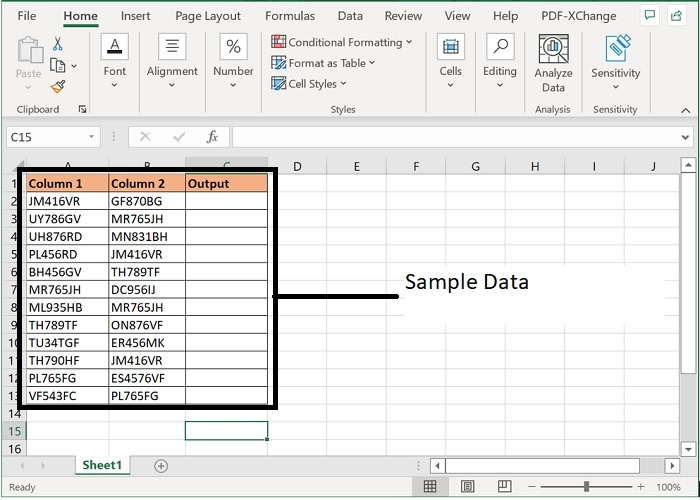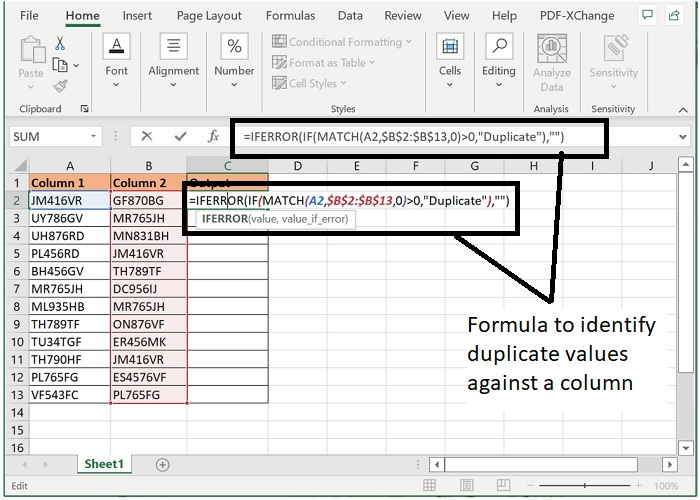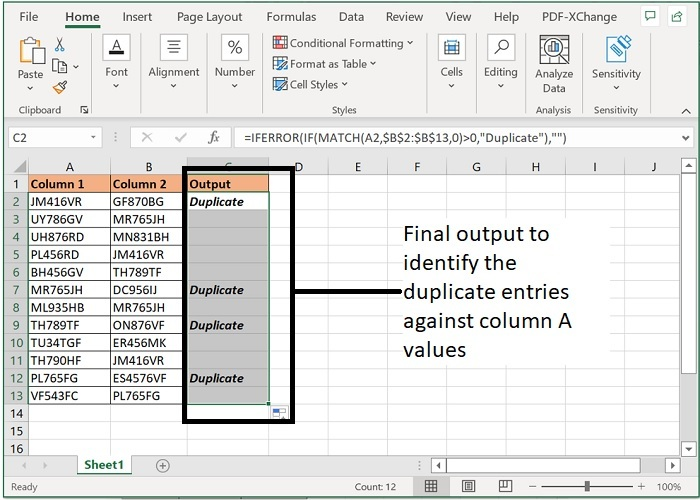# How to compare alphanumeric values in two columns in Excel?

Many times, we need to compare and identify the duplicate entries available in various columns of the excel data. Searching the duplicate entry of each value one by one is a tedious task. This can be achieved through a formula combination of excel sheet. Let’s see how the same can be achieved using the below steps.

## Compare alphanumeric values in two columns to find the same duplicates

Step 1: We have taken the following sample data for comparison.Step 2: Enter the formula in C2 cell as mentioned below. This formula will return Duplicate for those values which are equal or for those values which are duplicate.

=IFERROR(IF(MATCH(A2,$B$2:$B$13,0)>0,"Duplicate"),"")Step 3: Now, drag the formula till the last row of data and the final output will be as following.### Formula Syntax Description

Argument Description
IFERROR (value, value_if_error)

Value the argument that needs to be checked for errors.

Required Value_if_error the value to be returned if error occurs. Required

MATCH (lookup_value, lookup_array, [match_type])

Lookup value is the value that needs to be matched in look up array. Required

Look up array is the range of cells in which lookup value to be searched. Required

Match type specifies what type of match to be performed. 1 is for larger values, 0 stands for exact values and -1 stands for lesser values.

Note: In above formula, A2 and B2 are the two numbers that are being compared, duplicate is being returned against the values of column A.

## Conclusion

Hence using the above formula, two values can be compared for duplicity. One value can be compared with multiple range arrays as well. Using these steps, you can filter your dataset and create MIS reports as per the requirement. Keep learning excel.

Updated on: 29-Aug-2023

218 Views